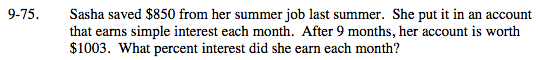### Home > MC2 > Chapter 9 > Lesson 9.3.1 > Problem9-75

9-75.Find the amount of money Sasha gained over the 9 months (the interest).

$1003 −$850 = \$153

Use the equation I = Prt, where I is the interest, P is the principle (original amount she had), r is the rate (percent interest per month), and t is the time (number of months that have passed).

153 = (850) r (9) Solve for r.

$r = \frac{153}{850 (9)}$

2% interest